# Finding the maximum height of a projectile

• Qube
In summary, the two solutions to the problem are depending on the assumption that the vertical component of the water's velocity will be zero when it hits the wall. If that assumption is incorrect, then the book takes the lower of the two heights that one finds after solving the system of equations.f

#### Qube

Gold Member
Homework Statement
Find the maximum height on the wall to which the firefighter can project water from his hose. The speed of the water from the hose is 65 ft/s. The height at which the hose is held is 3 feet.
Relevant Equations
All the kinematic equations for constant acceleration:

vf=vi+at
vf^2=vi^2-2a*s
sf=si+vi*t+0.5at^2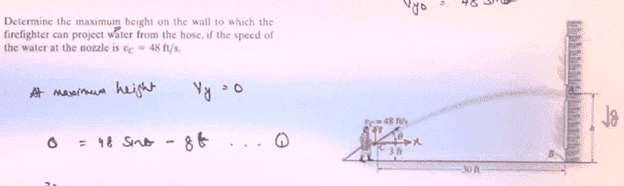Using the equations for constant acceleration, we can write the following set of equations for this problem: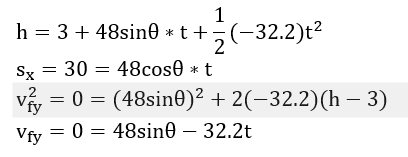We have the following known physical constraints: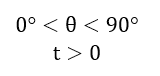Solving the above system of equations and constraints with a computer algebra system: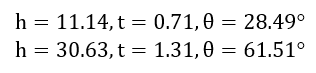So, all the solutions I found make physical sense. It's not as any of the solutions are saying that the firefighter is holding the hose backwards with respect to the wall or something.

My teacher said the answer is: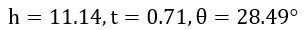And my teacher made no note of the other solution set.

Why is it that this problem, even when set up with the proper equations and reasonable physical constraints, yields two unique solution sets?

Why are you assuming that the vertical component of the water's velocity will be zero when it hits the wall?

•Qube
That's a great question, and from my discussions and research, I was told that this assumption is incorrect in this problem.

That being said, the book's solution manual makes that assumption, and so does my Dynamics professor: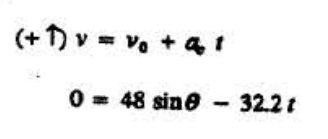But it seems that even if we take this assumption as valid (which it is not), the book chooses the lower of the two heights that one finds after solving the system of equations I presented.

Why is it that the book takes the lower of the two heights?

What should the takeaway be here? What is the pedagogial value of this problem?

Last edited:
That's a great question, and from my discussions and research, I was told that this assumption is incorrect in this problem.
I agree that that assumption is incorrect. Making that (incorrect) assumption turns the problem into solving for the angle of the hose so that when the water hits the wall it is at the maximum height of its trajectory.

Given that assumption, there are two solutions. Picking either is arbitrary. But if you wanted to imagine you are finding the maximum height (which you are not, given the mistaken assumption), why not pick the second solution?

What should the takeaway be here? What is the pedagogial value of this problem?
My advice: Solve the actual problem as stated. Find the maximum height on the wall that the water can reach.

Present that solution to your instructor and see what he says.

•Qube
My advice: Solve the actual problem as stated. Find the maximum height on the wall that the water can reach.

It seems that to solve the problem as stated would actually involve finding an equation relating the angle, theta, and the height on the wall, h. And then you would take the partial derivative of height equation with respect to theta to find the extrema.

Present that solution to your instructor and see what he says.

I'm currently awaiting a response :).

It seems that to solve the problem as stated would actually involve finding an equation relating the angle, theta, and the height on the wall, h. And then you would take the partial derivative of height equation with respect to theta to find the extrema.
Sounds good to me.

I'm currently awaiting a response :).I got a non-response response, so I guess I'll just chalk this up to YET another case of bottom-barrel textbook editors doing bottom-barrel work in writing questions and solutions. Book in question is Hibbeler's Dynamics, 14th edition. I used Hibbeler's Statics text last semester, and the professor warned me: there are lots of things that are just plain wrong in all of his textbooks.

Interesting puzzle.
Using the erroneous assumption that it coincides with the peak of the arc, I get the greater of the two solutions as 27.63 ft, rather less than your 30.63ft, but with the same 61.5 degree angle. That would be the solution to choose, not the lower one.
Using your correct approach I get 29.5ft, so only a little more. Angle 67.3 degrees.
In fact, keeping it entirely algebraic, the correct solution in general only beats the higher top-of-arc solution in a fourth order term.
Correct: ##\frac{v^2}{2g}-\frac{gx^2}{2v^2}##
Higher top-of-arc: ##\frac{v^2}{4g}(1+\sqrt{1-\frac{4g^2x^2}{v^2}})##

Last edited:
•Qube and Doc Al
I get the same results as @haruspex (which means it's correct).

And I know the frustration of poorly edited textbooks.

•Qube
Let me add to this discussion my take of what might be going on. First, I too confirm the results of @haruspex. What follows is a clarification for @Qube.

By eliminating time from the two kinematic equations, the equation referred to by OP in post #6 can be written as (I don't think I am giving anything away at this point)$$y=x \tan\theta-\frac{1}{2}g\left(\frac{x}{v_0\cos\theta}\right)^2$$With the substitutions ##u=\tan\theta## and ##\dfrac{1}{\cos^2\theta}=u^2+1##, this becomes$$y=xu-\frac{g(u^2+1)x^2}{2 v_0^2}.$$In most projectile problems the angle and hence ##u## is fixed and we have a parabola ##y(x)## where ##x## is the independent variable. Here the range ##x## is fixed, ##u## is the independent variable and we have a parabola ##y(u)##. The two parabolas have different values for ##y_{max}## unless (a) one is confused about what one is doing or (b) ##u^2>>1## in which case $$y\approx xu-\frac{gx^2u^2}{2 v_0^2}.$$In this approximation, the two parabolas have the same maximum value ##y_{max}=\dfrac{v_0^2}{2g}## at ##xu=x\tan\theta=v_0^2/g.## This is the exact expression for the maximum height when the projectile is shot straight up and is the limiting value in the "large angle approximation" regime, ##u^2=\tan^2\theta >>1##.

•Qube
Can we alternatively use energy methods to solve for the height of the water?

Can we alternatively use energy methods to solve for the height of the water?
The equation ##2ay=v_{fy}^2-v_{iy}^2## can be transformed into the work-energy theorem. If you multiiply both sides by ##\frac{m}{2}##, you have ##may=\frac{1}{2}mv_{fy}^2-\frac{1}{2}mv_{iy}^2##. With ##a=g##, you get ##mgy=K_f-K_i##. So in a sense you already have used energy methods except that this doesn't buy you much. You don't know ##v_{fy}## and we have already established that you cannot assume that it is zero.

•Qube
I don't suppose it will be of much interest to the math whizzes, but just for my own peace of mind, I plotted a few graphs.
FWIW? Here are a set of three which show me what's going on. (As usual when the algebra got messy, sorry, I just did an iterative numerical approximation.)
I did angles much closer to the optimum, but spread them out to show the peak at 30 jet.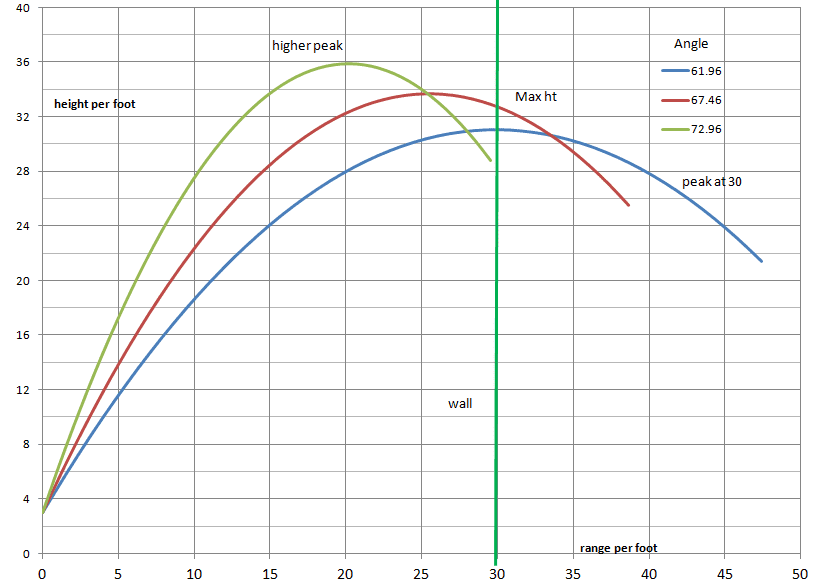••gmax137, Qube, Doc Al and 1 other person